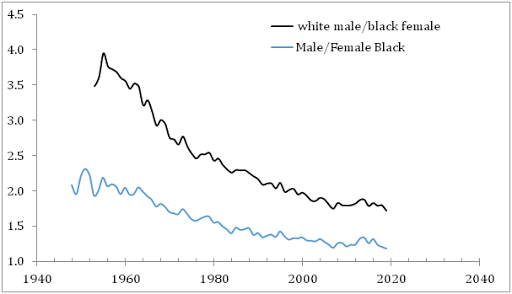## 10/24/14

### Long-term economic growth is linear

In this blog, we have discussed already [here and here] the incompatibility of real GDP data caused by the change in definition of the GDP deflator, dGDP, (in the USA - in 1977).  Figure 1 shows details of the deviation between the dGDP and the consumer price index, CPI, as expressed by the cumulative inflation rates. One can see that the CPI inflation rate is approximately equal to the rate of the GDP deflator change multiplied by a factor of 1.22 since 1978. This allows recovering the dGDP time series back in time using the strong statistical link between CPI and dGDP (1.22dGDP = CPI) , as shown in Figure 1.

This observation naturally leads to the assumption that real GDP in the United States is biased by the change in definition of the GDP deflator. (According to “Concepts and Methods of the U.S. NIPA” the growth rate of real GDP is the growth rate of nominal GDP reduced by the overall change in prices as expressed by the GDP deflator or the economy-wide price index.)  The Bureau of Economic Analysis warns economists that the real GDP time series is incompatible over time. What is the consequence of this bias to the long-term economic trends? One can correct real GDP time series for the definitional change and estimate the change in trend.  Figure 2 shows real GDP and real GDP per capita in the USA from 1929 to 2013. The latter time series has rather a linear trend since 1929 with Rsq. =0.97. The real GDP series deviates from the long term exponential trend since 2000 – the year then the rate of population growth fell below 1% per year.

In Figure 3, we correct real GDP per capita for the difference between CPI and dGDP after 1977 and compare the original and corrected time series. One can see that the corrected curve has Rsq.=0.99 and does not deviate from the long-term trend. Currently, the corrected growth rate is slightly below the long-term trend. (Obviously, we could correct the period before 1977 and obtain the same statistical result.  Statistically, the Solow model (constant returns to scale) implying the exponential GDP per capita growth is wrong.

Figure 1.  Cumulative rates of CPI and dGDP inflation, original and scaled by a factor of 1.2.

Figure 2. Real GDP and real GDP per capita in the USA from 1929 to 2013. The latter time series has rather a linear trend since 1929. The real GDP series deviates from exponential trend since 2000 – the year then the rate of population growth fell below 1% per year.

Figure 3. The real GDP per capita time series corrected for the difference between CPI and dGDP since 1978. Linear trend is obvious in the corrected time series. Currently, the growth rate is slightly below the long-term trend.

### The mean income gap between white males and black females grows during the democratic presidencies

Two days ago, we compared the mean income evolution of the white and black population and demonstrated that the difference did not change mu...